# 4 1 4 2 Quadratic Functions Vertex Form

• Slides: 114 -1 & 4 -2 Quadratic Functions: Vertex Form & Standard Form Today’s Objective: I can graph a quadratic function in vertex or standard form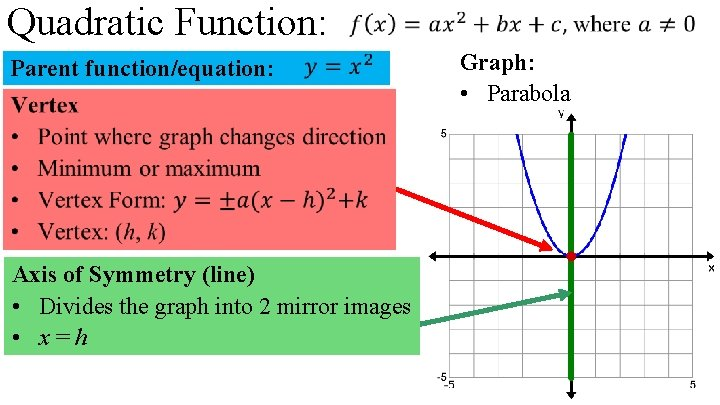Quadratic Function: Parent function/equation: Axis of Symmetry (line) • Divides the graph into 2 mirror images • x = h Graph: • Parabola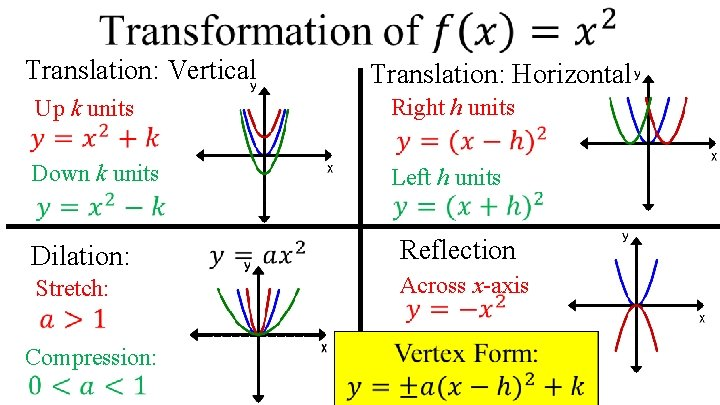Translation: Vertical Translation: Horizontal Right h units Up k units Down k units Left h units Dilation: Reflection Across x-axis Stretch: Compression: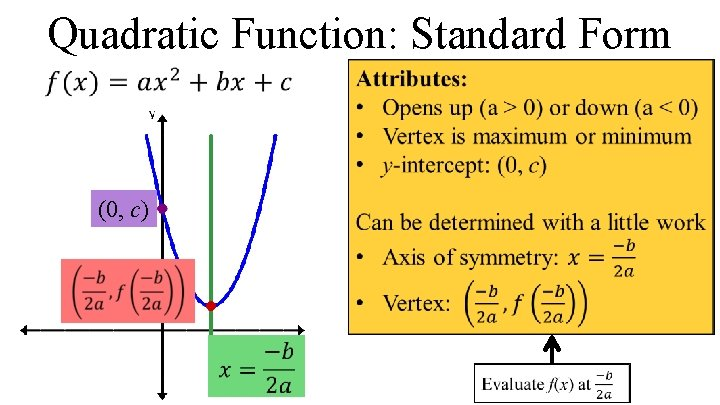Quadratic Function: Standard Form (0, c)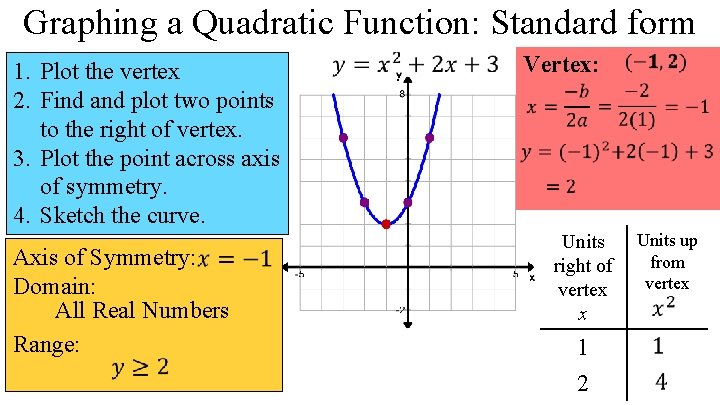Graphing a Quadratic Function: Standard form 1. Plot the vertex 2. Find and plot two points to the right of vertex. 3. Plot the point across axis of symmetry. 4. Sketch the curve. Axis of Symmetry: Domain: All Real Numbers Range: Vertex: Units up from right of vertex x 1 2Graphing a Quadratic Function: Standard form 1. Plot the vertex 2. Find and plot two points to the right of vertex. 3. Plot the point across axis of symmetry. 4. Sketch the curve. Axis of Symmetry: Domain: All Real Numbers Range: Vertex: Units up from right of vertex x 1 2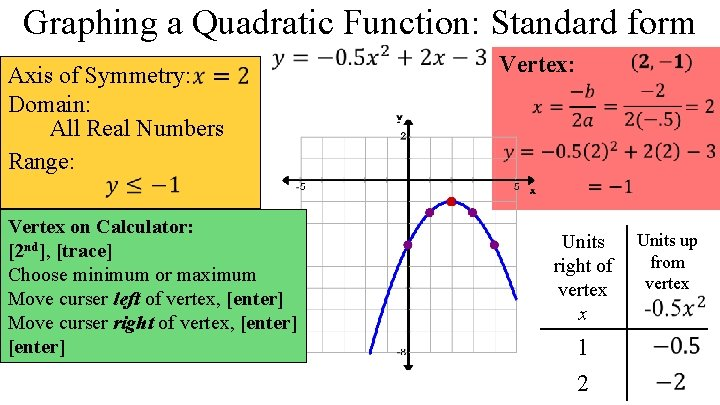Graphing a Quadratic Function: Standard form Axis of Symmetry: Domain: All Real Numbers Range: Vertex on Calculator: [2 nd], [trace] Choose minimum or maximum Move curser left of vertex, [enter] Move curser right of vertex, [enter] Vertex: Units up from right of vertex x 1 2Standard form to Vertex formBungee Jumping Maximum Arch height: p. 206: 9 -29 odds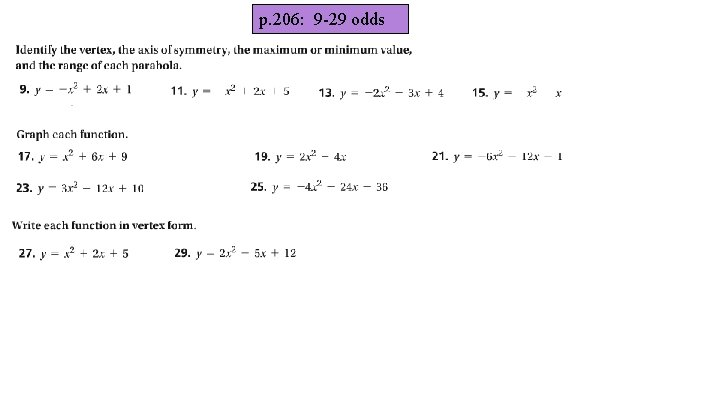p. 206: 9 -29 odds